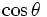# Tube of a curve

## Definition

Let$\gamma$ be a smooth curve (viz, a curve having a tangent at every point) in$\R^3$ and$r$ a length. The tube of radius$r$ about$\gamma$ is the union of the following circles:

For each point on the curve, choose the circle of radius$r$ about that point in the plane perpendicular to the tangent direction at that point.$r$ is termed the tube radius.

For a closed curve that does not intersect itself, we can choose a tube radius such that no two of these circles described above intersect.

Note that when the curve is a planar curve, viz it lies completely in a plane, the tube of that curve is symmetric about the plane.

## Structure of the tube

### Foliation of the tube

Given a curve, the tube of the curve comes with a natural foliation into circles. Thus, the tube of any curve is a cyclic surface. Moreover, it is a special kind of cyclic surface where all circles have equal radius.

### Special curves on the tube

There are four curves of special interest on the tube. These are:

• The inner rim of the tube is the curve comprising the tips of radial vectors from points on the original curve, that point along the inward normal.
• The outer rim of the tube is the curve comprising the tips of radial vectors from points on the original curve, that point along the outward normal.
• The top rim and bottom rim are curves comprising the tups of radial vectors in the binormal and reverse binormal directions

## Examples

### Tube of a straight line

The tube of a straight line is a right circular cylinder. The straight line is the axis of the cylinder.

For the straight line, there is no distinguished normal direction, hence there is no distinguished inner rim, outer rim, top rim or bottom rim. All straight lines on the cylinder that are parallel to the axis, qualify as rims.

Note that for any smooth open curve that is diffeomorphic to the real line, the tube about it is diffeomorphic to the right circular cylinder.

### Tube of a circle

The tube of a circle is a torus. The circle is the base circle of the torus.

Any smooth closed curve that is diffeomorphic to a circle, has a tube that is diffeomorphic to the torus.

## Symmetries of the tube and the curve

In general, any symmetry of the curve also gives a symmetry of the tube. There may be nontrivial symmetries of the tube which are trivial on the curve. For instance, for a planar curve, the reflection about its plane is a nontrivial symmetry of the tube that is trivial on the curve.

## Curvatures of the tube

At any point on the tube, the two principal directions are:

• The tangent to the foliation circle through that point. This circle lies in a cross-sectional plane, perpendicular to the tangent to the inner curve through the center.

The principal curvature in this direction is$1/r$

• The direction parallel to the tangent to the inner curve. This direction is perpendicular to the cross-sectional plane.

The principal curvature in this direction is$\cos \theta$ times the curvature of the inner curve at that point. Here$\theta$ is the angle made by the radial to that point, with the normal vector at the center of the circle.